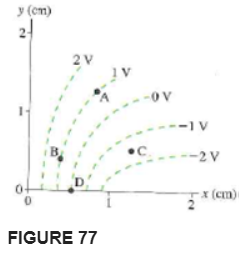# Problem: Figure 77 shows a series of equipotential curves.a. Is the electric field strength at point A larger than, smaller than, or equal to the field strength at point B? Explain. b. Is the electric field strength at point C larger than, smaller than, or equal to the field strength at point D? Explain. c. Determine the electric field E at point D. Express your answer as a magnitude and direction.

###### FREE Expert Solution

The electric field and electric potential are related by:

$\overline{){\mathbf{E}}{\mathbf{=}}\frac{\mathbf{∆}\mathbf{V}}{\mathbf{d}}}$

84% (145 ratings)###### Problem Details

Figure 77 shows a series of equipotential curves.

a. Is the electric field strength at point A larger than, smaller than, or equal to the field strength at point B? Explain.

b. Is the electric field strength at point C larger than, smaller than, or equal to the field strength at point D? Explain.

c. Determine the electric field E at point D. Express your answer as a magnitude and direction.Frequently Asked Questions

What scientific concept do you need to know in order to solve this problem?

Our tutors have indicated that to solve this problem you will need to apply the Equipotential Surfaces concept. You can view video lessons to learn Equipotential Surfaces. Or if you need more Equipotential Surfaces practice, you can also practice Equipotential Surfaces practice problems.